# Math Manipulatives: Calculators, Calculator Apps for Mobile Devices, and Related ResourcesMath Manipulatives contains three pages of resources:

Math Manipulatives (Page 1 of 3): Read a short essay about virtual manipulatives and their role in learning mathematics

Virtual Manipulatives on the Web (Page 2 of 3): Access our extensive list of virtual manipulatives on the web.Math Manipulatives (Page 3 of 3):## Calculators and PDA Resources Including Tutorials, Activities, Software Enhancements, and Apps for Mobile DevicesThere are many kinds of online and hand-held calculators.  Some are dedicated to specific professional fields; others have features beyond the capabilities of learners to use those, or features that learners might not be able to use on state standardized testing.  Some displays of online calculator input or output are easier to work with than others.  Therefore, educators should help learners choose those appropriate for the level of math they are learning, thus avoiding the potential problem of "teaching one way, but testing another way."

Consider the following.  A  graphing calculator is an essential tool for learners in mathematics courses such as algebra, trigonometry, advanced math, and calculus.  The visual display becomes a powerful tool for teaching and learning to show the link among conceptual, procedural, analytic, and investigative dimensions of learning mathematics.  When it comes to using a graphing calculator (or some scientific calculators with certain non-graphing features) on state standardized testing, there may be restrictions on use that educators should be aware of depending on grade level of the learner taking the exam.

What are the calculator policies for Common Core Assessments?Allowable calculators per the PARCC Calculator Policy (2012, updated 2017, p. 1) include:

• Grades 3-5: No calculators allowed, except for students with an approved calculator accommodation
• Grades 6-7: Four-function with square root and percentage functions
• Grade 8: Scientific calculators
• High school: Graphing calculators (with functionalities consistent with TI -84 or similar models).

Also see the Smarter Balanced Calculators: Powered by Desmos.

### Tutorials, Activities, and Software Enhancements

Manufacturers and technology organizations offer tutorials and manuals for elementary, financial, graphing, and scientific calculators.  Examples follow:

Casio Education training for Casio calculators.  Casio also has guided tours and product videos.  In Resources, educators will also find activities and sample questions.

Handheld Geometry "is for anyone who wants to use dynamic geometry to do mathematics on a handheld device, a graphic calculator, or the related computer software," according to site developer Nevil Hopley who is a math educator in Scotland.  Content is geared toward math typically taught to students ages 11 to 18: straight lines, circles, triangles, quadrilaterals, optimizing, loci, percentages, ratio, connections, statistics, spatial, sequences.  Video how-to's and notes accompany numerous graphic illustrations.

Hewlett-Packard calculators learning modules

Prentice Hall Graphing Calculator Help for TI-82, TI-83, TI-85, TI-86, TI-89, TI-92, HP48G, CFX-9850, Sharp EL9600C, and Casio FX2.

SimCalc MathWorlds software for TI-graphing calculators, computer, and TI-Navigator was developed by the University of Massachusetts-Dartmouth's James J. Kaput Center for Research and Innovation in STEM Education.  Animations, real life examples, narrative stories, and more are used to explain math concepts.

Sharp scientific calculators educational support

Texas Instruments Classroom Activities are free, standards-aligned, and designed for use with Texas Instrument graphing calculators.  You'll find activities for the TI-84 and MathNspire in various subjects such as middle grades math,  algebra 1, algebra 2, geometry, precalculus, calculus, and statistics.  There are sections called TI Code and Building Concepts in Mathematics, activities aligned to TEKS, CCSS, and more.  Tutorials are available for TI calculators.

### Online Calculators and PDA ResourcesNote: Several of the following resources are free for online use or download.

Accessible Calculators --this list provides information on the types of accessible calculators and potential sources.  It was developed at the Georgia Tech Research Corporation Center for Assistive Technology and Environmental Access.

Algebrahelp.com Calculator Index lists the online calculators provided at the site for solving and working with algebra equations, simplifying expressions, graphing functions, prime factoring numbers, operations with fractions, solving proportions, and so on.

Calc 3D is downloadable mathematical graph and charting software for geometry and statistics.  The calculator can do statistics, best fits, function plotting, polar and parametric plotting, integration. It handles vectors, matrices, complex numbers, quaternions, coordinates (Cartesian, polar, spherical, cylindrical), regular polygons, and intersections.  Find distances, volume, area, and more.  The software handles several languages, too.

CalculateWhat.com features a variety of free online calculators for just about anything, with a large emphasis on math calculators for algebra, geometry, statistics, and general math.  Each page also offers formula information and a brief background on each particular subject.  New calculators are added regularly.

Calculators On-Line Center features over 19,000 calculators for mathematics, statistics, science, and engineering.  Calculators for mathematics range from those suitable for basic mathematics through calculus and higher level mathematics.  Don't miss this vast collection of specialized calculators by topic from J. Martindale.

Calculators.org contains a collection of free online calculators on the Web organized by theme: savings, loans, debt, budget, automotive, credit cards, investing, mortgage, math, retirement, business, and health.  Math calculators from basic math to calculus are arranged by subject, topic, and level of difficulty.

Calculator Soup contains free online calculators, many of which also provide work and explanations to help understand the calculations.  They are grouped by categories: mathematics, financial (e.g., interest), and other (e.g., conversions).  Math includes calculators on fractions, general math, algebra, discrete math, geometry, plane and solid geometry, statistics, trigonometry, and word problems.

Coolmath Online Graphing Calculator is free and has all the common operators and functions expected in scientific calculators and graphing calculators for graphing functions.  Great alternative for students who forget their own handheld graphing calculator, such as the TI-83.

Derivative Calculator is free and finds derivatives of functions.  "The Derivative Calculator supports computing first, second, …, fifth derivatives as well as differentiating functions with many variables (partial derivatives), implicit differentiation and calculating roots/zeros. You can also check your answers! Interactive graphs/plots help visualize and better understand the functions" (About section).

HOT!: Desmos Graphing Calculator is free from Desmos.com and compatible with any computer or tablet.  Examples of graphs possible are also at Chrome.Google, where you can also launch the app.  It is full featured, and comes with support for using features.  Multiple graphs can be placed on top of one another.  You can show Cartesian and polar coordinates and zoom in and out of plots. Graphs can be saved or printed and axes labeled in terms of "pi" for typical trig graphs.  There are options for displays in radians and degrees.  Points can be plotted at users choice and tables of values shown on a graph can be displayed.  You can use set notation, graph inequalities, explore transformations, evaluate equations, and more.  3D graphing is an option.  There is a projector mode for class use.  This is a winner for math educators!

EasyCalculation has a series of free online calculators set up as they relate to specific math concepts for number, algebra, statistics, geometry, trigonometry, calculus, and more.  There are also some tutorials on the math concepts related to those calculations.

eCalc is "a free online calculator that supports many advanced features including unit conversion, equation solving, and even complex-number math.  The calculator is designed to work directly in your browser and requires no special plug-ins."  There is a basic calculator and a scientific calculator, the latter of which includes trig, log, and exponential functions, and decimals to fractions.  Both have large keys for easy input.  eCalc is not a graphing calculator.  However, it's definitely worth investigation.  You can also download the calculator.

e-Tutor online graphing calculator is free and can be used to graph simple to advanced equations.  Multiple equations can be shown on a single graph.

GCalc is a free online graphing calculator. "GCalc is designed to provide a basic, easy-to-use, well-balanced set of graphing functionality for algebra, precalculus, calculus and beyond."

Good Calculators has over 200 free online calculators grouped into 24 categories for easy selection.  These are useful in school settings, business, and for math in daily life.  Insight into how calculations are derived is provided where possible.  Calculators are by John Sanders and Andrew Stacy.

GraphCalc is a Windows 2D/3D graphing calculator.  Download it for free.  The developers call it "an all-in-one solution to everything from everyday arithmetic to statistical analysis, from betas to Booleans, from cubes to calculus, from decimals to derivatives. GraphCalc combines all the features of a professional mathematics package with the simplicity of an easy to learn windows interface. It provides user-friendly help and tutorials to guide you through the easy and fun process of mastering GraphCalc."  A Linux version is also available.

InstaCalc is a free online calculator that can interpret natural language expressions and equations.  It also includes instructions for getting started. Results are displayed instantly, and can be shared.  Perform basic math, convert units of measure and currency, use variables and rows, create charts similar to what you'd do with spreadsheets, work with trigonometry, logarithms, programming tools, and more.  You can add notes and embed your calculations in your own web pages, too.  It's amazing.

Integral Calculator is free and finds integrals and antiderivatives of functions.  It shows you the full step by step integration.  "All common integration techniques and even special functions are supported. The Integral Calculator supports definite and indefinite integrals (antiderivatives) as well as integrating functions with many variables. You can also check your answers! Interactive graphs/plots help visualize and better understand the functions" (About section).

HOT!: Math Portal Online Calculators and Solvers by mathematician Miloš Petrović contains multiple math calculators and solvers grouped into 17 categories.  Use this site for problems including polynomial equations, rational expressions, systems of equations, vectors, matrices, complex numbers, plane and analytic geometry, statistics, trigonometry, calculus (limits, derivatives, integrals), sequences, series, financial, numbers, and more.  Per the description, "These calculators can help you with basic and advanced problems in high school and college math.  All of them are capable of performing exact computations.  Also, almost every of them will generate a step by step explanation."  The step by step explanations are exceptionally valuable for learners.  There are three other math sections on the site: formulas, tests and lessons.  Math lessons relate to algebra, calculus, analytic geometry, and linear algebra, and also include solved problems and examples for students to solve.

Math is Fun: Matrix Calculator enables the user to work with matrices up to 10 x 10.  Operations include addition, subtraction, multiplication, finding a determinant, an inverse, squaring a matrix, and more.

MatrixCalc.org: Matrix Calculator helps the user to find the determinant of a matrix, the rank, raise a matrix to a power, find sum and multiplication of matrices, calculate the inverse matrix.  Enter the matrix elements and click a button.  You can also solve systems of equations using matrices.

HOT!: Meta-Calculator is a free online calculator, which the developer (Vernon Morris, a math and computer science educator) indicated "can indeed do most of the important things that the TI's can do" (personal communication, July 27, 2013).  It's a graphing calculator, scientific calculator, matrix calculator, and statistics calculator.  A blog is included at the site.  Key features:

• Graph up to seven equations, including equations like circles and hyperbolas; find intersections.  Work with common functions such as square root, trig, logarithmic, and inverse functions.  Choose radian or degree mode, set domain and range, produce table of values, plot points.  You can also graph polar equations.  Save the image of your graph to your computer.
• The scientific calculator performs all the basic functions you'd expect and there are advanced features.  You can find the least common multiple, permutations, and combinations.  There's a linear equations solver that lets you input up to six equations with either two or three variables and the solver will calculate the solutions.  Store calculations using the memory button.
• Add, subtract, multiply, and transpose matrices.  Calculate the determinant or inverse of a matrix.
• Statistical calculations include finding quartiles, mean, median, mode, standard deviation, correlation coefficients, and almost any kind of regression (e.g., linear, quadratic, cubic, exponential, power, log, natural log).  Regressions can be graphed.  Also perform T-tests (paired and unpaired, 1 or 2-tailed).

Microsoft Mathematics 4.0 is a FREE download! It includes a full-featured graphing calculator that’s designed to work just like a handheld calculator.  You can plot in 2D and 3D; there's step-by-step equation solving, and additional math tools to help you solve triangles, convert from one system of units to another, and solve systems of equations.  There's a library of equations and formulas and ink handwriting support.  This is a great teaching and learning tool, which also comes with a teaching guide.

Omnicalculator.com has over 100 online calculators designed "to make your life easier."  They are in multiple categories, such as business, construction, conversion, finance, fitness, health, math, physics, and statistics.  Each has a specific purpose.  Several come with additional explanations on the concept.

Online Statistics Calculators:  There are five statistics calculators available from Alcula.com: measures of central tendency and dispersion, box and whisker plots, linear regression, correlation coefficients, and scatter-plots.  Other online calculators for math are also available.

• Smart Math Calculator computes the result of any math expression as you type. There are over 20 math functions and scientific constants. A free version of this calculator is available for use online or as a download to your computer.
• Graphing Calculator 3D is a tool for plotting 2D and 3D functions. It comes with several features.  Among those are plotting regular and parametric equations and coordinates tables.  Use Cartesian/Polar coordinates in 2D; 3D features Cartesian/Cylindrical/Spherical coordinates. You can plot inequalities in 2D and 3D. 2D and 3D have an animation and variable slider.  Rotate, translate, and zoom graphs; 3D graphs can be shaded.  A free version of this calculator is also available for use online or as a download to your computer.
• Runiter Company (Canada) also produces problem solver calculators for statistics and calculus, and a more advanced graphing calculator for 2D and 3D.

Statistics Calculators at DanielSoper.com are free for the research community with 29 categories available.

HOT!: Symbolab Equation Calculator "allows users to search and solve equations, formulas and expressions using mathematical symbols and scientific notations as well as text. Symbolab solves math problems." It provides automated step by step solutions to algebraic, trigonometry, and calculus topics covering from middle school math through college.  Note: This can be a valuable tool.  Algebra includes solving equations (e.g., linear, quadratic, absolute value, log, radical, etc.), inequalities, systems of equations, line equations, basic operations.  For trig, you can prove identities and solve trig equations in degrees or radians.  For calculus, work with limits, integrals, derivatives (including implicit), derivative applications (including tangents and normals to curves), and series.  Examples with step by step solutions are provided.  Results of queries can be saved, including notes, and then shared with others.

Talking Calculator is an onscreen full-function talking calculator that can be used with or without a screen reader.  "Every button and edit area talks. It is easy to use with large keys and contrasting colors. It has three display areas, so when the user adds a series of numbers, the total is always displayed, even as you are entering a new number, while always displaying any numbers in memory." Further, "it displays your entries and results as you work. It actually displays the equation so that you can easily see or hear your last process. When students are required to show their work, they can simply cut and paste the steps into a document."  Perform simple and basic operations.  A free download is available.

The Calculator Site by Alastair Hazell contains multiple free online calculators and converters.  There are four sections: financial calculators, unit conversions, health and math calculators, and miscellaneous calculators.  HOT!: Math educators will appreciate the three fractions calculators.  The first will add, subtract, multiply or divide fractions, including mixed numbers, and display the answer in fraction and number form.  The second will simplify fractions, using the greatest common factor, and show the result as a fraction and number.  Both also show the explanations on how answers were derived and if the number form is a repeating decimal, a bar is shown over digits that repeat--a very unique feature to such calculators.  The third converts a decimal to a fraction.  A unique feature of this latter is that it also accommodates converting repeating decimals to fractions by also entering the number of decimal places that repeat.  The output shows the repeating decimal with a bar over the digits that repeat and the equivalent fraction.

Unit Conversion from PDFConverter.com is a free series of unit converters with multiple options for converting mass, time zones, area, angle, length, volume, pressure, temperature, and data storage.  Quick and easy to use.

Zona Land's graphics calculator, EZ Graph, enables you to graph almost any polynomial, rational, exponential, logarithmic, or trigonometric function.

### Calculator Apps for Mobile DevicesCalcMadeEasy Pro is designed for iPhone and iPad and available in iTunes for \$1.99.  It's designed to enable "financial calculations/decision making, scientific/mathematical calculations, unit conversions and automatic notes." CalcMadeEasy free scientific calculator with auto notes for Mac is also available.Ezy Graph by KGiants is for iPad and iPhone.  This free app for high school learners and above is available in iTunes.  It supports most graphing functions and comes with advanced graph analysis (e.g., domain, zeros, max/min/inflection points of graphs), multiple graphs on a set of axes, customized graphs with color and line style, and trace mode for coordinates of points.  You can share, save, and print graphs.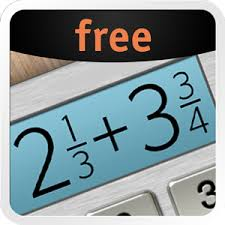Fraction Calculator Plus by Digitalchemy, LLC is a free app compatible for use with iPhone, iPod touch, and iPad.  Anyone working with fractions to add, subtract, multiply, or divide can benefit.  Output is displayed in lowest fraction terms and in decimal format.Kalkulilo (scientific calculator) by Alexander Clauss is a free calculator app for iPhone and iPad.  It comes with several modes.  The scientific mode includes the usual functions and operations such as basic arithmetic, powers, roots, logarithms, trigonometry, and so on.  A bit/integer mode offers logic operations and calculations in 8, 16, 32, or 64 bits.  The statistics mode enables calculations of mean, median, mode, quartile values, variance, standard deviation, range, and so on.Mathpix features solving and graphing math using pictures.  This app for Android and iOS (iPad, iPhone, and iPod touch) devices can be used to convert handwritten or printed equations into digital format by taking a picture of the equation.  Mathpix uses Desmos for graphing in 2D and 3D.  Descriptions indicate support for arithmetic, fractions, algebraic equations, roots, logarithms, trigonometry, integrals, derivatives, and infinite series, matrices, and piecewise functions.  You can also export equations to a pdf.  There is a free version and in-app purchases for full access.Math 42 by Cogeon GmbH features a "symbolic algebra system" on this calculator app designed for iPhone and iPad.  It is appropriate for learners in middle school, high school, and college.  Per the description, it supports not just answers, but step-by-step solutions.  The free version includes the following:

• Simplifications and transformations (Signs, combining, factorizing, binomial decomposition, …)
• Fractions (adding, reducing, multiplying, simplifying, GCD, LCM, …)
• Logarithms and trigonometric functions (rad/deg)
• Linear, fractional, quadratic equations and systems of equations (linear and non-linear)
• Polynomial long division
• Derivation (sums, exponents, products, quotients, chain, powers, roots, trigonometry, …)
• Graphical Curve Sketching (Derivation, roots, extremes, inflection points, poles, asymptotical functions, intersection points)

The subscription-based premium version of Math 42 offers additional features.MegaPro Calculator 5000 by Chris Ternoey
Price: Free
Target: Elementary, Ages 6-8
Info: Young children learn the concepts of addition and subtraction of large numbers and place value.  Visualization of the regrouping process is a key feature.  Demos available.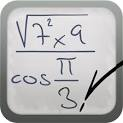MyScript Calculator by MyScript is a free app for iPad and iPhone, available on iTunes.  Enter your expression using symbols and numbers in your own handwriting and this app converts it to digital text and performs the calculation.  The calculator supports basic operations, powers, roots, exponentials, logarithms (ln and log) trig, percents, absolute value, factorials, using brackets, and includes constants (pi, e, phi).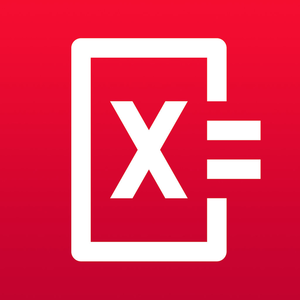Photomath, developed by Microblink, reads and solves math problems by using the camera on your mobile device (iPhone, iPad, or Android) to capture the problem.  It recognizes handwriting, provides step-by-step instructions and explanations, allows for keyboard problem entry.  Per its description: "Photomath supports arithmetics, integers, fractions, decimal numbers, roots, algebraic expressions, linear equations and inequalities, quadratic equations and inequalities, absolute equations and inequalities, systems of equations, logarithms, trigonometry, exponential and logarithmic functions, derivatives and integrals."  The app is free, but does offer in-app purchases.  Graphing has been added in version 4.0.1.Pomegranate Software Calculators and apps for mobile devices include those for iPad, iPhone, Android, and Mac. For example, see the calculator Solve.  It also supports graphing 2D and 3D functions using the x, y and θ variables.Symbolic Calculator by Voxeloid Kft. is for iPhone or iPad, and available for \$2.99 in iTunes.  "Symbolic Calculator is capable of helping with any kind of math problems ranging from basic arithmetics to university-level advanced math. It offers all the advantages of an advanced graphing/scientific calculator mixed with the convenience of modern mobile apps."Talking Scientific Calculator by Adam Croser is designed for iPad and iPhone (\$4.99).  It "has large colorful buttons, optional high contrast, full VoiceOver support, and unique to this calculator; the option to use speech for answers, buttons names and formulas."  Supports entry and display of Braille on supported devices.  The accessibility features on this app also make it highly suitable for use by learners with disabilities.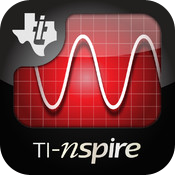TI-Nspire and TI-Nspire CAS (Computer Algebra System) graphing calculator apps for iPad are from Texas Instruments. These apps enable "comprehensive graphing, data entry and analysis, statistical modeling and calculating functionality" (iTunes Description section).Trigonometry Pro HD by voi nguyen can be used on an iPad.  It is available in iTunes for a couple of dollars.  This "Interactive Trigonometry Calculator contains 13 Modules: Geometry, The Right Triangular Shapes, 30/60 Degrees Triangular Shapes, 45 Degrees Triangular Shapes, Oblique Triangular Shapes, Trigonometry/Geometry Equations, The Ultimate Units Converter, Animated Sin/Cos/Tan Graphs Functions, Interactive Central Angle, Interactive Unit Circle, Circle Functions, Lines, Point & Slopes Plot Functions and the bonus module is: The Arabic Numerals System."

Here's a bit of history for you!

From Slates, to Slide Rules and Software!Does anyone remember the slide rule?  "Throughout American history, teachers and parents have used objects--from colonial--era slates to modern electronic calculators--to help students master abstract mathematical concepts," according to The Smithsonian Institute, which has posted a highly informative display called Slates, Slide Rules, and Software: Teaching Math in America.  Read developments in math education and teaching with manipulatives from the Early Republic, to the Cold War, and Information Age.  Additional resources are provided.

Did you know?"The slide rule has a long and distinguished ancestry … from William Oughtred in 1622 to the Apollo missions to the moon ... a span of three and a half centuries … it was used to perform design calculations for virtually all the major structures built on this earth during that long period of our history … an amazing legacy for something so mechanically simple" (Source: The Oughtred Society, History of the Slide Rule).  Read more about it and other calculating instruments at the Oughtred Society.

The ways that people have been computing dates back thousands of years.  The tools have changed of course.  See the Chronological Calculator Timeline, which dates clay tablets being used for commercial transactions by Sumerians around 4000-12000 BC.  See key events to the present time.

Calculating Firsts: A Visual History of Calculators traces how calculating machines have developed over the years from the first adding machine in 1623 to the first color-display graphing calculator in 2010.

Get the scoop on the history of the internet, a timeline of computer history, and learn about the people involved in making computers what they are today.  Visit the Computer History Museum.Get a little of the history of PDAs in a 3-part series at The Mobile Spoon (2009).

Did you know that slates are alive and well?

Consider the modern updated slate: the netbook and iPad, for example.Can you guess the origin of the radical sign from the picture?  Get a little of the history of the radical sign (√ ) and see when other math symbols were first introduced.

What's the history of irrational numbers?  In Making sense of irrational numbers, a TED-Ed lesson video (about 5 minutes), educator Ganesh Pai "describes the history and math behind irrational numbers."  She begins telling the viewer, "Like many heroes of Greek myths, the philosopher Hippasus [8 BC] was rumored to have been mortally punished by the gods. But what was his crime? Did he murder guests or disrupt a sacred ritual? No, Hippasus's transgression was mathematically proving the hitherto unprovable."  He is credited with the discovery of irrational numbers, thus revolutionizing mathematics.

Here's just two:The irrational number Pi (π) is the ratio of the circumference of a circle to its diameter.  It is often used in mathematics approximated as 3.14, but its value has been calculated to a length of 31 trillion digits!  See the BBC news report of March 14, 2019: Emma Haruka Iwao smashes pi world record with Google help.  Per this news, "The calculation required 170TB of data (for comparison, 200,000 music tracks take up 1TB) and took 25 virtual machines 121 days to complete."

Numberphile produced a video, Mile of Pi, which shows pi printed to a million digits on paper and shown over a mile long on an airport runway.  "Pi" has been expressed as a continued fraction, one of which is the following.  Notice its beautiful pattern: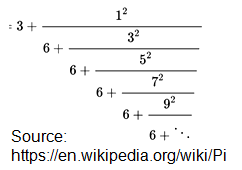Learn more about the history of pi.  Also visit the Joy of Pi.  See the interactive demonstrations Approximating Pi at PBS Nova or Computing Pi at NCTM's Illuminations.  You will experience how the Greek mathematician Archimedes determined a theoretical approach to the calculation of pi using a circle and finding perimeters of inscribed and circumscribed polygons.  This took place around 250 BC and the demonstration is still useful.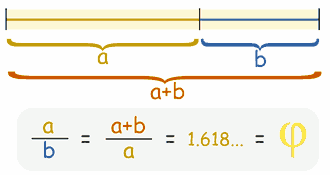The golden number, Phi (φ) 1.618..., is an irrational number, like Pi (π).  It is found in many places, as in properties of the human body, in plants, DNA, the solar system, art and architecture (its uses date back to the ancient Egyptians and Greeks), the stock market, the Bible and theology (think of the book Da Vinci Code), population growth, and so on.  Learn more about it at GoldenNumber.net.  Math is Fun also provides learners with an easy to understand explanation of the Golden Ratio, the Golden Section, and Fibonacci numbers.

Use the Golden Ratio Calculator at Omnicalculator.com to find two lengths that would compute to give the golden ratio.

#### References

PARCC. (2012, July).  Approved Calculator Policy. Updated August 2017.  Retrieved from https://www.isbe.net/Documents/Calculator-policy2018.pdfBack to top | Math Manipulatives: Page  1  |   2  |   3See related topics:  Math Resources and Standardized Test Preparation.